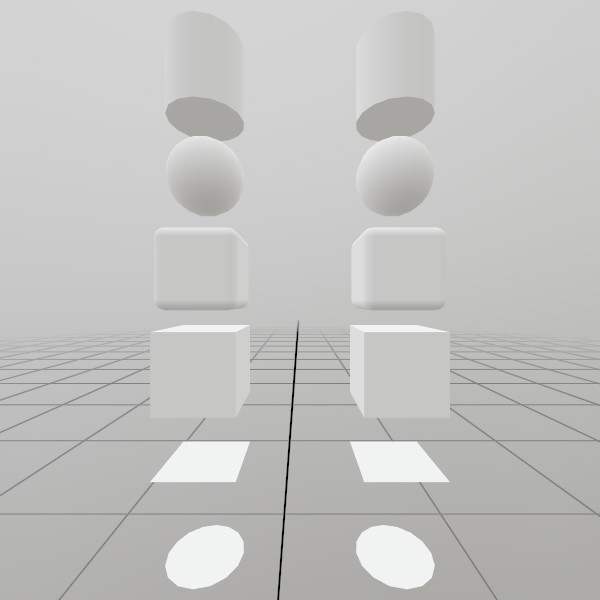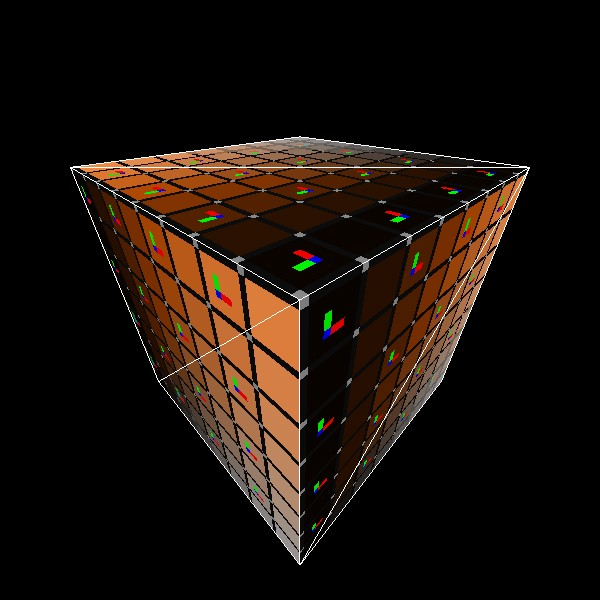# Mesh.GenerateCube

``````static Mesh GenerateCube(Vec3 dimensions, int subdivisions)
``````

Generates a flat-shaded cube mesh, pre-sized to the given dimensions. UV coordinates are projected flat on each face, 0,0 -> 1,1.

NOTE: This generates a completely new Mesh asset on the GPU, and is best done during ‘initialization’ of your app/scene. You may also be interested in using the pre-generated `Mesh.Cube` asset if it already meets your needs.

Vec3 dimensions How large is this cube on each axis, in meters?
int subdivisions Use this to add extra slices of vertices across the cube’s faces. This can be useful for some types of vertex-based effects !
RETURNS: Mesh A flat-shaded cube mesh, pre-sized to the given dimensions.

## Examples

### Generating a Mesh and ModelHere’s a quick example of generating a mesh! You can store it in just a Mesh, or you can attach it to a Model for easier rendering later on.

``````Mesh  cubeMesh  = Mesh.GenerateCube(Vec3.One * 0.4f);
Model cubeModel = Model.FromMesh(cubeMesh, Default.Material);
``````

Drawing both a Mesh and a Model generated this way is reasonably simple, here’s a short example! For the Mesh, you’ll need to create your own material, we just loaded up the default Material here.

``````// Call this code every Step

Matrix cubeTransform = Matrix.T(-.5f, -.5f, 0);
cubeMesh.Draw(Default.Material, cubeTransform);

cubeTransform = Matrix.T(.5f, -.5f, 0);
cubeModel.Draw(cubeTransform);
``````

### UV and Face layout

Here’s a test image that illustrates how this mesh’s geometry is laid out.``````meshCube = Mesh.GenerateCube(Vec3.One);
``````

Found an issue with these docs, or have some additional questions? Create an Issue on Github!